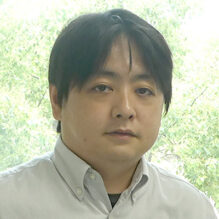# Sho Tanimoto

Priority Organization for Innovation and Excellence, Kumamoto UniversityAssociate Professor*Profile is at the time of the award.

2019Inamori Research GrantsScience & Engineering

Research topics
Research on Rational and Integral points via Higher Dimensional Algebraic Geometry
Keyword
Summary
Diophantine equations, i.e., polynomial equations with integer coefficients, and their integral and rational solutions have been studied since the age of Greece. As a circle is defined by one polynomial equation, a system of polynomial equations defines a geometric object in the space which we call as an algebraic variety. Then rational solutions can be considered as points on that geometric object and we call them as rational points.

I study arithmetic properties of rational points using techniques from higher dimensional algebraic geometry such as the minimal model program (Mori's theory). On the other hand I also apply ideas from arithmetic geometry to problems in algebraic geometry. I am very fascinated by the deep connection between geometry and number theory. I enjoy exploring new possibilities of mathematics and I hope to further contribute to the development of mathematics.

## Message from recipient

I am very honored to receive Inamori research grant. I continue to explore new possibilities of mathematics and hope to contribute to the development of mathematics.

## Find other recipients

Science & Engineering